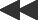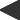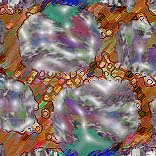basis: barnsley-fractal z(0) = pixel; z(n+1) = (z-1) * c if real(z) >= 0, else z(n+1) = (z+1) * c two parameters: real and imaginary parts of c.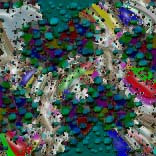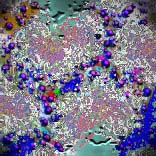Michael Barnsley has written a fascinating college-level text, "Fractals Everywhere," on fractal geometry and its graphic applications. In it, he applies the principle of the Mandelbrot and Julia sets to more general functions of two complex variables.